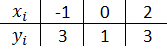Home MathLab Matlab Exercises

# Matlab Exercises

It’s a great time to hit the road running. We have got enough Matlab lessons to start handing you some nice Matlab challenges to help you to assess yourself.

Here are some Matlab exercises.

Related Post: Free Matlab Alternative

## Matlab Exercises

### Exercise 1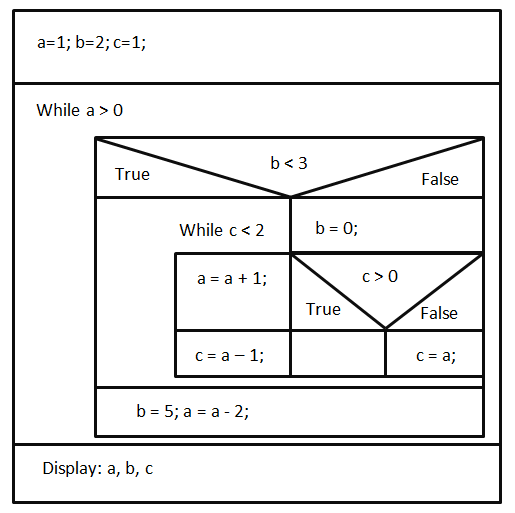1. Write a Matlab code corresponding to the above structogram.

2. What are the value of a, b and c at the end of this program?

### Exercise 2

Analyze the following code

[php]x=1;
y=2;
z=3;
for i=1:3
x=2*x;
while x<5
y=y+1;
z=z*x;
x=y;
end
end
fprintf(‘x=%i \n’,x);
fprintf(‘y=%i \n’,y);
fprintf(‘z=%i \n’,z);[/php]

1. Without using neither a calculator nor your computer, compute the values of x, y and z at the end of this program.

2. Now check your result using Matlab. (Simply copy the code, paste in Matlab and run it)

### Exercise 3

Let’s consider a cone. Write a Matlab program that computes the volume of a cone. Here is the formula you should be using.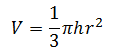Where r is the radius of the base and h is the height. Write a Matlab program that finds the radius of a cone. The program should first check that r and h are both positive. The precision of the result should be at most 3 decimal digit.

### Exercise 4

Let’s consider the following function saved in the file recursive.m

[php]function[y]=recursive(x,y)
if (x>y)
y=recursive(y,2)-1;
else
y=3;
end[/php]

1.  What will be the result of y=recursive(9,7)?

2. What will be the result of y=recursive(9,11)?

### Exercise 5

The following Matlab programs contain some elementary programming mistakes. Find the mistake and suggest a solution to each or them.

(a)

[php]function[d]=pol2(h)
d=0;
i=0;
while i<h
d=d+1;
end[/php]

(b)

[php]
function[l]=pol3(arr)
for i=1:length(arr)
diff(i)=arr(i)-arr(i+1);
end[/php]

(c)

if r=0
fprintf(‘The number is zero’);
else
fprintf(‘The number is negative’);[/php]

### Exercise 6

Write a Matlab function that computes the following sum while requesting the value of x and n from the user.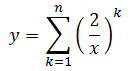To call the function, the user should use the following syntax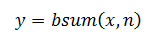### Exercise 7

Consider the following Matlab program

[php]value=[2 5 7 -2 8 6 4 3];
Data=[4 5 3 7];
r=4;
s=1;
t=7;
x=top(value, r, t);
y=top(value, s, r);
z=value(8);
fprintf(‘x=%i \n’,x);
fprintf(‘y=%i \n’,y);
fprintf(‘z=%i \n’,z);[/php]

The function top defines the following way

[php]function[g]=top[ele, p, k]
g=ele(p)-ele(k);[/php]

Without using your computer, find the value of x, y and z at the end of this program.

### Exercise 8

We say a n × n matrix A is symmetric when for all i, j=1,…,n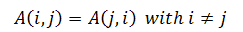Let’s consider the following matrices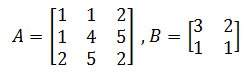A is symmetric but B is not

Write a Matlab function that will test if a matrix is symmetric or not. The function will return the number “1” if the matrix is symmetric and “0” if it is not.

To call the function you should use the following syntax

Symmetric=SymmetricMatrix(A)

### Exercise 9

Solve the following equations using Matlab

(a)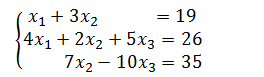(b)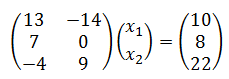### Exercise 10

Graph the equation manually and plot the graph in Matlab to check your result having the following information